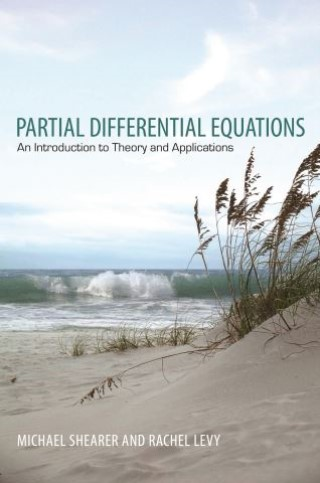## Partial Differential Equations• Description
• Author
• Info
• Reviews

### Description

An accessible yet rigorous introduction to partial differential equations

This textbook provides beginning graduate students and advanced undergraduates with an accessible introduction to the rich subject of partial differential equations (PDEs). It presents a rigorous and clear explanation of the more elementary theoretical aspects of PDEs, while also drawing connections to deeper analysis and applications. The book serves as a needed bridge between basic undergraduate texts and more advanced books that require a significant background in functional analysis.

Topics include first order equations and the method of characteristics, second order linear equations, wave and heat equations, Laplace and Poisson equations, and separation of variables. The book also covers fundamental solutions, Green's functions and distributions, beginning functional analysis applied to elliptic PDEs, traveling wave solutions of selected parabolic PDEs, and scalar conservation laws and systems of hyperbolic PDEs.

• Provides an accessible yet rigorous introduction to partial differential equations
• Draws connections to advanced topics in analysis
• Covers applications to continuum mechanics
• An electronic solutions manual is available only to professors
• An online illustration package is available to professors